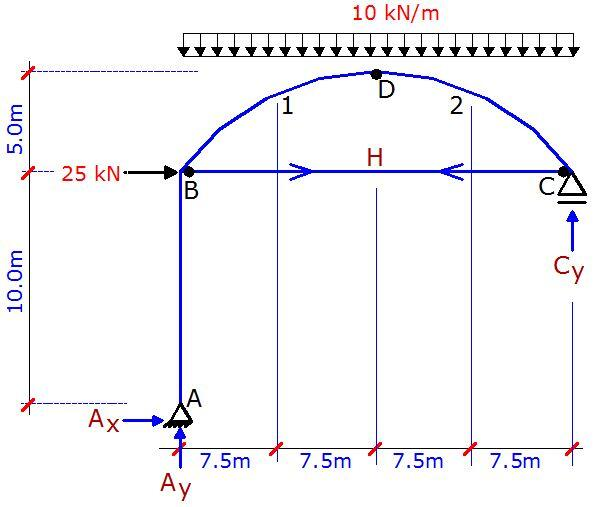# Analysis of Arch-Frame Structure with Tie Element: A solved ExampleAn arch is supported on frame with different levels of supports as shown above. The frame is supported on a hinge at support A and a roller at support C. A tie beam is used to connect node B and support C. Plot the bending moment, shear force, and axial force diagram die to the externally applied load.

Solution

Support Reactions

∑MC = 0
30Ay – 10Ax  –  (10 × 302)/2 = 0
30Ay – 10Ax = 4500 ————- (1)

∑FX = 0
Ax + 25 = 0
Ax = -25 kN (pointing in the reverse of assumed direction)

Plugging the value of Ax into equation (1);
30Ay – 10(-25) = 4500
30Ay = 4250
Ay = 141.667 kN

∑MA = 0
30Cy  – (25 × 10) – (10 × 302)/2 = 0
30Cy = 4750
Cy = 158.333 kN

∑MDR = 0

15Cy –  (H × 5) – (10 × 152)/2 = 0
15(158.333) – 5H – (10 × 152)/2 = 0
– 5H + 1250 = 0
H = 250 kN

Geometric Properties of the Arch Section

The ordinate of the arch at any given horizontal length section is given by;

y = [4yc (Lx – x2)] / L2

Where yc is the height of the crown of the arch
y = [(4 × 5) × (30x – x2)]/302 = (2/3)x – (x2/45)

dy/dx = y’ = 2/3 – 2x/45

At x = 0; y = 0
y’ = 2/3 – 0/8 = 0.667
sin⁡θ = y’/√(1 + y’2) = 1/√(1 + 0.6672) = 0.5546
cos⁡θ = 1/√(1 + y’2) = 1/√(1 + 0.6672) = 0.8319

At x = 7.5m;
y = (2/3)x – (x2/45) = (0.667 × 7.5) – (7.52/45) = 3.75m
y’ = 2/3 – 2(7.5)/45 = 0.333
sin⁡θ = y’/√(1 + y’2) = 0.333/√(1 + 0.3332) = 0.43159
cos⁡θ = 1/√(1 + y’2) = 1/√(1 + 0.3332) = 0.9487

At x = 15 m;
y = (2/3)x – (x2/45) = (0.667 × 15) – (152/45) = 5.00m
y’ = 2/3 – 2(15)/45 = 0
sin⁡θ = y’/√(1 + y’2) = 0/√(1 + 02) = 0
cos⁡θ = 1/√(1 + y’2) = 1/√(1 + 02) = 1.0

At x = 22.5m;
y = (2/3)x – (x2/45) = (0.667 × 22.5) – (22.52/45) = 3.75m
y’ = 2/3 – 2(22.5)/45 = -0.333
sin⁡θ = y’/√(1 + y’2) = -0.333/√(1 + 0.3332) = -0.43159
cos⁡θ = 1/√(1 + y’2) = 1/√(1 + 0.3332) = 0.9487

At x = 30m; y = 0
y’ = 2/3 – 2(30)/45 = -0.667
sin⁡θ = y’/√(1 + y’2) = -0.667/√(1 + 0.6672) = -0.5546
cos⁡θ = 1/√(1 + y’2) = 1/√(1 + 0.6672) = 0.8319

Internal Stresses in the Arch Structure
Bending Moment
MA = 0
MB= (25 × 10)  = 250 kN.m
MBUP = (25 × 10)  = 250 kN.m
M1 = (141.667 × 7.5) + (25 × 13.75) – (25 × 3.75) – (10 × 7.52)/2 – (250 × 3.75) = 93.7525 kN.m
MD = (141.667 × 15) + (25 × 15) – (25 × 5) – (10 × 152)/2 – (250 × 5) = 0

Coming from the right
M2 = (158.333 × 3.75) – (250 × 5) – (10 × 122)/2 = -31.252 kN.m
MC = 0

Shear force

Section A – B (Column)
Q– Ax = 0
Q–  25 = 0
Q– QBB  = 25  kN

Qi = ∑V cos⁡θ – ∑H sin⁡θ (for arch section)

Note that the horizontal forces of 25 kN will eliminate each other;

QBUP =  (141.667 × 0.8319) – (250 × 0.5546) = -20.797 kN
Q1R = Q1L = [141.667  – (10 × 7.5)] × 0.9487  – (250 × 0.43159) = -44.651 kN
QD =  [141.667 – (10 × 15)] × 1.0 – (250 × 0) = -8.333 kN
Q2R = Q2L =  [141.667 – (10 × 22.5)] × 0.9487 – (250 × –0.43159) = 28.839 kN
QC = [141.667 – (10 × 30)] × 0.8319 – (250 × -0.5546) = 6.932 kN

Axial force
Member AB = 141.667 kN (compression)
Member BC = 250 kN (Tension)

For the arch section;
Ni = -∑V sin⁡θ – ∑H cos⁡θ
NBUP = -(141.667 × 0.5546) – (250 × 0.8319) = -286.543 KN (Compression)
N1L = -[141.667 – (10 × 7.5)] × 0.43159 – (250 × 0.9487) = -265.848 KN
ND = -[141.667 – (10 × 15)] × 0 – (250 × 1) = -250 KN (Compression)
N2L = N3R = -[141.667 – (10 × 22.5)] × -0.43159 – (250 × 0.9487) = -273.141 KN
NC = -[141.667 – (10 × 30)] × -0.5546 – (250 × 0.8319) = -295.786 KN

Internal Stresses Diagram

Thank you for visiting Structville.